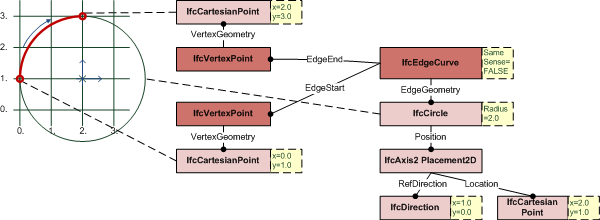# IFC 4.3.x development

## 8.20.3.5 IfcEdgeCurve

### Change log

#### IFC4

 modifications attributes SameSense definition Changed from boolean to IfcBoolean

### 8.20.3.5.1 Semantic definitions at the entity

An IfcEdgeCurve defines two vertices being connected topologically including the geometric representation of the connection.

NOTE  The topology is used to trim the geometry of the edge. There is no need to geometrically trim the edge to match the topology.EXAMPLE  Figure 1 illustrates an example where the edge geometry is given by an unbounded curve, here IfcCircle. The bounds are provided by the EdgeStart and EdgeEnd, the topological direction of the IfcEdgeCurve opposes the direction of the IfcCircle by SameSense = FALSE. Figure 1 — Edge curve

NOTE  Definition according to ISO/CD 10303-42:1992
An edge curve is a special subtype of edge which has its geometry fully defined. The geometry is defined by associating the edge with a curve which may be unbounded. As the topological and geometric directions may be opposed, an indicator (same sense) is used to identify whether the edge and curve directions agree or are opposed. The Boolean value indicates whether the curve direction agrees with (TRUE) or is in the opposite direction (FALSE) to the edge direction. Any geometry associated with the vertices of the edge shall be consistent with the edge geometry. Multiple edges can reference the same curve.

NOTE  Entity adapted from edge_curve defined in ISO 10303-42.

HISTORY  New entity in IFC2x.

Informal Propositions:

1. The domain of the edge curve is formally defined to be the domain of its edge geometry as trimmed by the vertices. This domain does not include the vertices.
2. An edge curve has non-zero finite extent.
3. An edge curve is a manifold.
4. An edge curve is arcwise connected.
5. The edge start is not a part of the edge domain.
6. The edge end is not a part of the edge domain.
7. Vertex geometry shall be consistent with edge geometry.

### 8.20.3.5.1 Attributes

# Attribute Type Description
IfcRepresentationItem
LayerAssignmentSET [0:1] OF IfcPresentationLayerAssignment FOR AssignedItemsAssignment of the representation item to a single or multiple layer(s). The LayerAssignments can override a LayerAssignments of the IfcRepresentation it is used within the list of Items.

> IFC2x3 CHANGE  The inverse attribute LayerAssignments has been added.

> IFC4 CHANGE  The inverse attribute LayerAssignment has been restricted to max 1. Upward compatibility for file based exchange is guaranteed.
StyledByItem SET [0:1] OF IfcStyledItem FOR Item Reference to the IfcStyledItem that provides presentation information to the representation, e.g. a curve style, including colour and thickness to a geometric curve.

> IFC2x3 CHANGE  The inverse attribute StyledByItem has been added.
IfcTopologicalRepresentationItem
IfcEdge
1 EdgeStart IfcVertex Start point (vertex) of the edge.
2 EdgeEnd IfcVertex End point (vertex) of the edge. The same vertex can be used for both EdgeStart and EdgeEnd.
IfcEdgeCurve
3 EdgeGeometry IfcCurve The curve which defines the shape and spatial location of the edge. This curve may be unbounded and is implicitly trimmed by the vertices of the edge; this defines the edge domain. Multiple edges can reference the same curve.
4 SameSense IfcBoolean This logical flag indicates whether (TRUE), or not (FALSE) the senses of the edge and the curve defining the edge geometry are the same. The sense of an edge is from the edge start vertex to the edge end vertex; the sense of a curve is in the direction of increasing parameter.

### 8.20.3.5.3 Formal representations

```ENTITY IfcEdgeCurve
SUBTYPE OF (IfcEdge);
EdgeGeometry : IfcCurve;
SameSense : IfcBoolean;
END_ENTITY;```